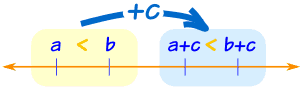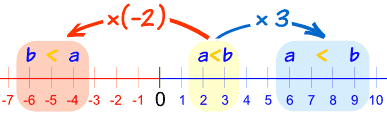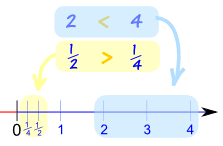# Properties of Inequalities

Inequality tells us about the relative size of two values.

(You might like to read a gentle Introduction to Inequalities first)

## The 4 Inequalities

Symbol
Words
Example
>
greater than
x+3 > 2
<
less than
7x < 28
greater than or equal to
5 ≥ x−1
less than or equal to
2y+1 ≤ 7The symbol "points at" the smaller value

## Properties

Inequalities have properties ... all with special names!

Here we list each one, with examples.

Note: the values a, b and c we use below are Real Numbers.

## Transitive Property

When we link up inequalities in order, we can "jump over" the middle inequality.If a < b and b < c, then a < c

Likewise:

If a > b and b > c, then a > c

### Example:

• If Alex is older than Billy and
• Billy is older than Carol,

then Alex must be older than Carol also!

## Reversal Property

We can swap a and b over, if we make sure the symbol still "points at" the smaller value.

• If a > b then b < a
• If a < b then b > a

Example: Alex is older than Billy, so Billy is younger than Alex

## Law of Trichotomy

The "Law of Trichotomy" says that only one of the following is true:It makes sense, right? a must be either less than b or equal to b or greater than b. It must be one of those, and only one of those.

### Example: Alex Has More Money Than Billy

We could write it like this:

a > b

So we also know that:

• Alex does not have less money than Billy (not a<b)
• Alex does not have the same amount of money as Billy (not a=b)

(Of course!)

## Addition and Subtraction

Adding c to both sides of an inequality just shifts everything along, and the inequality stays the same.If a < b, then a + c < b + c

### Example: Alex has less money than Billy.

If both Alex and Billy get \$3 more, then Alex will still have less money than Billy.

Likewise:

• If a < b, then a − c < b − c
• If a > b, then a + c > b + c, and
• If a > b, then a − c > b − c

So adding (or subtracting) the same value to both a and b will not change the inequality

## Multiplication and Division

When we multiply both a and b by a positive number, the inequality stays the same.

But when we multiply both a and b by a negative number, the inequality swaps over!Notice that a<b becomes b<a after multiplying by (-2)
But the inequality stays the same when multiplying by +3

Here are the rules:

• If a < b, and c is positive, then ac < bc
• If a < b, and c is negative, then ac > bc (inequality swaps over!)

A "positive" example:

Example: Alex's score of 3 is lower than Billy's score of 7.

a < b

If both Alex and Billy manage to double their scores (×2), Alex's score will still be lower than Billy's score.

2a < 2b

But when multiplying by a negative the opposite happens:

But if the scores become minuses, then Alex loses 3 points and Billy loses 7 points

So Alex has now done better than Billy!

−a > −b

### Why does multiplying by a negative reverse the sign?

Well, just look at the number line!

For example, from −3 to −7 is a decrease, but from 3 to 7 is an increase.Notice that−7 < −3
but+7 > +3

So the inequality sign reverses (from < to >)

## Additive Inverse

As we just saw, putting minuses in front of a and b changes the direction of the inequality. This is called the "Additive Inverse":

• If a < b then −a > −b
• If a > b then −a < −b

This is really the same as multiplying by (-1), and that is why it changes direction.

Example: Alex has more money than Billy, and so Alex is ahead.

But a new law says "all your money is now a debt you must repay with hard work"

So now Alex is worse off than Billy.

## Multiplicative InverseTaking the reciprocal (1/value) of both a and b can change the direction of the inequality.

When a and b are both positive or both negative:

• If a < b then 1/a > 1/b
• If a > b then 1/a < 1/b

### Example: Alex and Billy both complete a journey of 12 kilometers.

Alex runs at 6 km/h and Billy walks at 4 km/h.

Alex’s speed is greater than Billy’s speed

6 > 4

But Alex’s time is less than Billy’s time:

12/6 < 12/4

2 hours < 3 hours

But when either a or b is negative (not both) the direction stays the same:

• If a < b then 1/a < 1/b
• If a > b then 1/a > 1/b

## Non-Negative Property of Squares

A square of a number is greater than or equal to zero:

a2 ≥ 0

### Example:

• (3)2 = 9
• (−3)2 = 9
• (0)2 = 0

Always greater than (or equal to) zero

## Square Root Property

Taking a square root will not change the inequality (but only when both a and b are greater than or equal to zero).

If a ≤ b then √a ≤ √b
(for a,b ≥ 0)

### Example: a=4, b=9

• 4 ≤ 9 so √4 ≤ √9